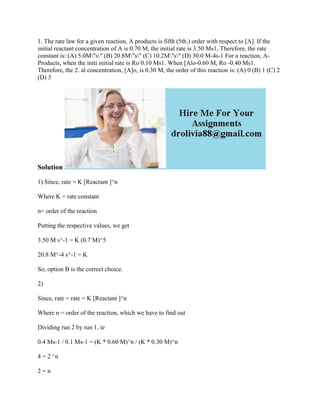Seu SlideShare está sendo baixado. ×

# 1- The rate law for a given reaction- A products is fifth (5th-) order.docx

Anúncio
Anúncio
Anúncio
Anúncio
Anúncio
Anúncio
Anúncio
Anúncio
Anúncio
Anúncio
AnúncioCarregando em…3
×

1 de 2 Anúncio

# 1- The rate law for a given reaction- A products is fifth (5th-) order.docx

1. The rate law for a given reaction, A products is fifth (5th.) order with respect to [A]. If the initial reactant concentration of A is 0.70 M, the initial rate is 3.50 Ms1, Therefore, the rate constant is: (A) 5.0M\"s\" (B) 20.8M\"s\" (C) 10.2M\"s\" (D) 30.0 M-4s-1 For a reaction, A-Products, when the initi initial rate is Ro 0.10 Ms1. When [Alo-0.60 M, Ro -0.40 Ms1. Therefore, the 2. al concentration, [A]o, is 0.30 M, the order of this reaction is: (A) 0 (B) 1 (C) 2 (D) 3
Solution
1) Since, rate = K [Reactant ]^n
Where K = rate constant
n= order of the reaction
Putting the respective values, we get
3.50 M s^-1 = K (0.7 M)^5
20.8 M^-4 s^-1 = K
So, option B is the correct choice.
2)
Since, rate = rate = K [Reactant ]^n
Where n = order of the reaction, which we have to find out
Dividing run 2 by run 1, ie
0.4 Ms-1 / 0.1 Ms-1 = (K * 0.60 M)^n / (K * 0.30 M)^n
4 = 2 ^n
2 = n
So, option C is the correct choice.
.

1. The rate law for a given reaction, A products is fifth (5th.) order with respect to [A]. If the initial reactant concentration of A is 0.70 M, the initial rate is 3.50 Ms1, Therefore, the rate constant is: (A) 5.0M\"s\" (B) 20.8M\"s\" (C) 10.2M\"s\" (D) 30.0 M-4s-1 For a reaction, A-Products, when the initi initial rate is Ro 0.10 Ms1. When [Alo-0.60 M, Ro -0.40 Ms1. Therefore, the 2. al concentration, [A]o, is 0.30 M, the order of this reaction is: (A) 0 (B) 1 (C) 2 (D) 3
Solution
1) Since, rate = K [Reactant ]^n
Where K = rate constant
n= order of the reaction
Putting the respective values, we get
3.50 M s^-1 = K (0.7 M)^5
20.8 M^-4 s^-1 = K
So, option B is the correct choice.
2)
Since, rate = rate = K [Reactant ]^n
Where n = order of the reaction, which we have to find out
Dividing run 2 by run 1, ie
0.4 Ms-1 / 0.1 Ms-1 = (K * 0.60 M)^n / (K * 0.30 M)^n
4 = 2 ^n
2 = n
So, option C is the correct choice.
.

Anúncio
Anúncio

### 1- The rate law for a given reaction- A products is fifth (5th-) order.docx

1. 1. 1. The rate law for a given reaction, A products is fifth (5th.) order with respect to [A]. If the initial reactant concentration of A is 0.70 M, the initial rate is 3.50 Ms1, Therefore, the rate constant is: (A) 5.0M"s" (B) 20.8M"s" (C) 10.2M"s" (D) 30.0 M-4s-1 For a reaction, A- Products, when the initi initial rate is Ro 0.10 Ms1. When [Alo-0.60 M, Ro -0.40 Ms1. Therefore, the 2. al concentration, [A]o, is 0.30 M, the order of this reaction is: (A) 0 (B) 1 (C) 2 (D) 3 Solution 1) Since, rate = K [Reactant ]^n Where K = rate constant n= order of the reaction Putting the respective values, we get 3.50 M s^-1 = K (0.7 M)^5 20.8 M^-4 s^-1 = K So, option B is the correct choice. 2) Since, rate = rate = K [Reactant ]^n Where n = order of the reaction, which we have to find out Dividing run 2 by run 1, ie 0.4 Ms-1 / 0.1 Ms-1 = (K * 0.60 M)^n / (K * 0.30 M)^n 4 = 2 ^n 2 = n
2. 2. So, option C is the correct choice.